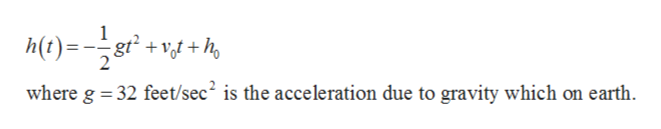A projectile is fired vertically upward from a height of 175175feet above the ground, with an initial velocity of 18001800ft/sec. Recall that projectiles are modeled by the function h(t)=−16t2+v0t+y0h(t)=−16t2+v0t+y0. Write a quadratic equation to model the projectile's height h(t)h(t)in feet above the ground after t seconds.

Question

A projectile is fired vertically upward from a height of
175
175
feet above the ground, with an initial velocity of
1800
1800
ft/sec. Recall that projectiles are modeled by the function
h(t)=−16
t
2
+
v
0
t+
y
0
h(t)=−16t2+v0t+y0
. Write a quadratic equation to model the projectile's height
h(t)
h(t)
in feet above the ground after t seconds.

Step 1

Consider the given data.

Step 2

The height h of a projectile above the ground after t seconds is given byhelp_outlineImage Transcriptionclosewhere g 32 feet/sec2 is the acceleration due to gravity which on earth fullscreen
Step 3

Therefore, the projectile...

Want to see the full answer?

See Solution

Want to see this answer and more?

Our solutions are written by experts, many with advanced degrees, and available 24/7

See Solution
Tagged in

Algebra